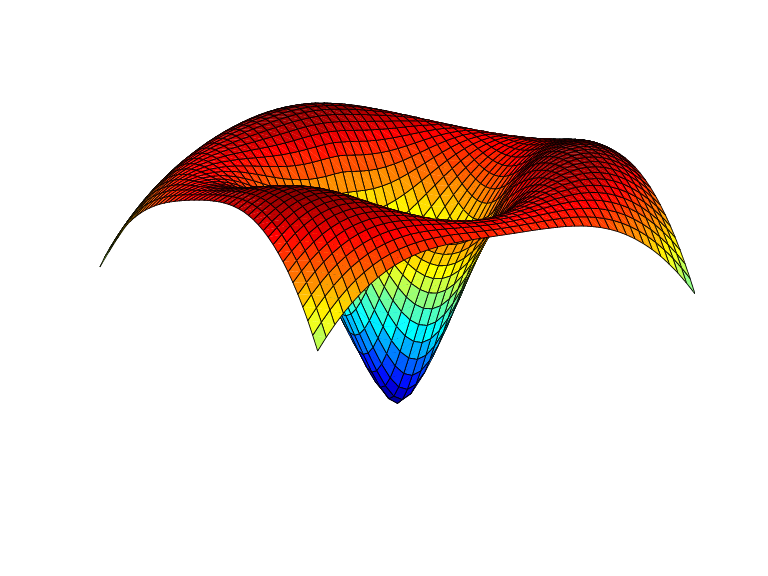stcol

Scattered translates collocation matrix

Syntax

colmat = stcol(centers,x,type)
colmat = stcol(...,'tr')

Description

colmat = stcol(centers,x,type) is the matrix whose (i,j)th entry is

with the bivariate functions ψj and the number n depending on the centers and the character vector or string scalar type, as detailed in the description of stmak.

centers and x must be matrices with the same number of rows.

The default for type is the character vector 'tp', and for this default, n equals size(centers,2), and the functions ψj are given by

with ψ the thin-plate spline basis function

$\psi \left(x\right)={|x|}^{2}\mathrm{log}{|x|}^{2}$

and with |x| denoting the Euclidean norm of the vector x.

Note

See stmak for a description of other possible values for type.

The matrix colmat is the coefficient matrix in the linear system

that the coefficients aj of the function f = Σjajψj must satisfy in order that f interpolate the value yi at the site x(:,i), all i.

colmat = stcol(...,'tr') returns the transpose of the matrix returned by stcol(...).

Examples

Example 1. The following evaluates and plots the function

$f\left(x\right)=\psi \left(x-{c}_{1}\right)+\psi \left(x-{c}_{2}\right)+\psi \left(x-{c}_{3}\right)-3.5\psi \left(x\right)$

on a regular mesh, with ψ the above thin-plate basis function, and with c1, c2, c3 three points on the unit circle; see the figure below.

a = [0,2/3*pi,4/3*pi]; centers = [cos(a), 0; sin(a), 0];
[xx,yy] = ndgrid(linspace(-2,2,45));
xy = [xx(:) yy(:)].';
coefs = [1 1 1 -3.5];
zz = reshape( coefs*stcol(centers,xy,'tr') , size(xx));
surf(xx,yy,zz), view([240,15]), axis offExample 2. The following also evaluates, on the same mesh, and plots the length of the gradient of the function in Example 1.

zz = reshape( sqrt(...
([coefs,0]*stcol(centers,xy,'tp10','tr')).^2 + ...
([coefs,0]*stcol(centers,xy,'tr','tp01')).^2),
size(xx));
figure, surf(xx,yy,zz), view([220,-15]), axis off